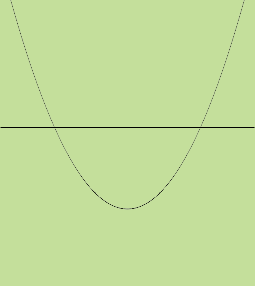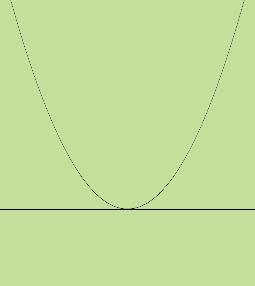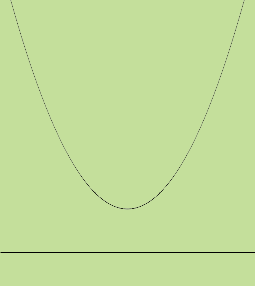SEARCH HOMEMath Central Quandaries & QueriesQuestion from Henry, a student: how many times does the graph of y=2xsquared -2x + 3 intersect the x-axis?Hi Henry,

I know that the graph of y = 2x2 -2x + 3 is a parabola and since the coefficient of x2 is positive (it's 2) the parabola opens upwards. Hence I know the graph looks likeThe question is "Where is the X-axis?"

 Is the axis above the vertex so the graph intersects the x-axis twice and the equation 2x2 -2x + 3 = 0 has two real roots?Does the axis pass through the vertex so the graph intersects the x-axis once and the equation 2x2 -2x + 3 = 0 has one real root?Is the axis below the vertex so the graph does not intersect the x-axis and the equation 2x2 -2x + 3 = 0 has no real roots?To decode which is the case solve the equation 2x2 -2x + 3 = 0, using the general quadratic if necessary, and decide how many real roots it has.

PennyMath Central is supported by the University of Regina and The Pacific Institute for the Mathematical Sciences.